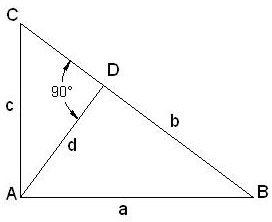# Math Help: Pythagoras Theorem and Theorem Proofs

Page content

Around 2530 years ago, Pythagoras first created the Pythagorean Theorem. A simple Pythagorean Theorem proof is making a pyramid with a perfect square or rectangular base.

## The Theorem

The square of the hypotenuse of a right angled triangle is equal to the sum of squares of other two sides.

See the attached snap of the triangle below (Click on it to enlarge):

So, as per Pythagoras theorem we can write that:

(BC)2 = (AB)2 + (AC)2

If we consider the side AB as a, AC as c and BC as b then we can write the Pythagorean Theorem as

b2=a2+ c2

Now you are in a position to calculate the length of any one side of a right angled triangle, if the lengths of the other two sides are given.

## Practice Problems

Solve the following examples. Assume c is the hypotenuse of a right angled triangle and a, b are the other two sides of the same triangle:

a = 3; b = 4; c=?

a=5; c= 13; b=?

a=8; b=15; c=?

a= 9; c=41; b=?

## Pythagorean Theorem ProofPythagoras' Theorem has more than 300 proofs. The simplest proof of the theorem is based on the similar triangles concept:

• Take the triangle ABC with AB=a, AC=c and BC=b.
• Drop a line from A to D which is perpendicular to BC.
• Triangle ACD is similar to the triangle ABC as:

Angle ADC = Angle CAB = 90 degree

Angle ACD = Angle ACB

Side AC is common for both the triangle

So, we can write from similar triangle principle:

c / b = DC / c

c2 = b X DC …………….eqn.1

• Again, triangle ABD and triangle ABC are similar because:

Angle ADB = Angle CAB = 90 degree

Angle ABD = Angle ABC

Side AB is common for both triangles.

So, we can write:

a / b = BD / a

a2 = b X BD …………….eqn.2

• From eqn.1 and eqn.2 we can write:

c2 +a2 = (b X DC) + (b X BD)

= b X (DC + BD)

= b X BC(as, BC consists of DC and BD)

= b X b(as, we already assume BC=b)

= b2

## Real Life Examples

• Making a perfect rectangular basketball and volleyball court.
• Measuring the height of ramp.
• Calculating distance between two points if co-ordinates of the points are given.

## Pythagoras Triples

Pythagoras triples are sets of three integer numbers which follows Pythagoras' Theorem. For example, take 3, 4, 5.

Remember the Pythagoras theorem (b2=a2+ c2).

Now, if you take a=3, c=4 then from the theorem b will be equal to 5. There are many such sets of integers like this: 5, 12,13; 17, 24, 25; 9, 40, 41 etc.

People have been using these triples even before human beings learned to write.